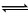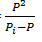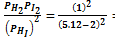Courses

# Removal / Addition Of Inert Gas At Equilibrium - Chemical Equilibrium Chemistry Notes | EduRev

## Physical Chemistry

Created by: Asf Institute

## Chemistry : Removal / Addition Of Inert Gas At Equilibrium - Chemical Equilibrium Chemistry Notes | EduRev

The document Removal / Addition Of Inert Gas At Equilibrium - Chemical Equilibrium Chemistry Notes | EduRev is a part of the Chemistry Course Physical Chemistry.
All you need of Chemistry at this link: Chemistry

Removal / Addition of inert gas at equilibrium

At constant volume:

Addition / removal of inert gas have no effect at equilibrium at constant volume.

At constant pressure:

If inert gas is added then to maintain the pressure constant, volume is increase.

Hence equilibrium will shift in the direction in which larger no. of moles of gas is formed.

(i)         Δng> 0

Reaction will shift in forward direction.

(ii)        Δng< 0

Reaction will shift in backward direction.

(iii)       Δng = 0, No effect

Simultaneous Equilibrium

Series Equilibrium:

When product of Initial reaction acts as reactants in further reactions.

A(S)B(g) + C(g)

Pi–P     Pi                   Kp1= Pi(Pi – P)

B(g)D(g) + E(g)

Pi – P               P          P                     Kp2=Parallel Equilibrium:

A(S)B(g) + C(g)

P1+P2     P1= (P1 + P2) P1

X(S)B(g) + D(g)

P1+P2     P2= P2(P1 + P2)

When two different reactions gives the same products, called parallel equilibrium.

Example:        NH4I(s)NH3(g) + HI(g)                       KP1 = 16

2 HI(g)H2(g) + I2 (g)

Pressure of Hydrogen at equilibrium is 1 atm. Calculate the value of KP2.

Solution:         NH4I(s)NH3(g) + HI(g)

Pi – 2        Pi

2HI(g)H2(g) + I2(g)

Pi – 2             1            1

Kp1 = (Pi – 2) (Pi)

Pi = 5.12

KP2 == 0.103

Offer running on EduRev: Apply code STAYHOME200 to get INR 200 off on our premium plan EduRev Infinity!

## Physical Chemistry

77 videos|83 docs|32 tests

,

,

,

,

,

,

,

,

,

,

,

,

,

,

,

,

,

,

,

,

,

;